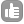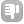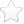1What is the energy difference between the anti-parallel and parallel states of the hydrogen nucleus in a 1.5T and a 3T magnetic field and what are the units of energy measured in? asked Aug 25 '09 at 13:43Grum 16Evgeny Fadeev 577●1 is that your homework :)? - Evgeny Fadeev (Aug 25 '09 at 17:28)

### The question has been closed for the following reason "the question is answered, right answer was accepted" by Evgeny Fadeev close date 2009-09-21 10:04:570For a given state ``````E = -hbar gamma m B m is one of (-1/2;1/2) proton spin states gamma - proton gyromagnetic ratio in 1/(Tesla*s) hbar - reduced Planck constant in m2kg/s `````` Difference between two states of a proton will be ``````|delta E| = hbar gamma B `````` Substitute values into the formula and get delta E in Joules or convert to other energy units. answered Aug 25 '09 at 17:25Evgeny Fadeev 577●1

Tags:

×7
×3
×2

Asked: Aug 25 '09 at 13:43

Seen: 5,027 times

Last updated: Nov 18 '09 at 14:28